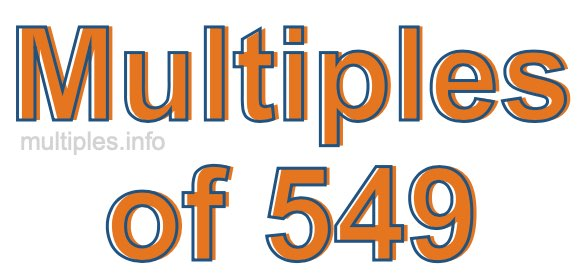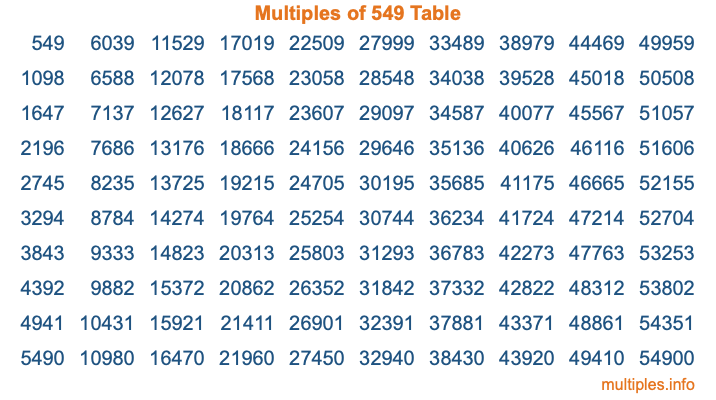Multiples of 549Welcome to the Multiples of 549 page. Here we will first teach you everything you will ever need to know about the multiples of 549, and then give you a study guide summary of everything we taught you to make sure you remember it all. Use this page to look up facts and learn information about the multiples of 549. This page will make you a multiples of five hundred forty-nine expert!

Definition of Multiples of 549
Multiples of 549 are all the numbers that when divided by 549 equal an integer. Each of the multiples of 549 are called a multiple. A multiple of 549 is created by multiplying 549 by an integer.

Therefore, to create a list of multiples of 549, you start with 1 multiplied by 549, then 2 multiplied by 549, then 3 multiplied by 549, and so on for as long as you want. Thus, the list of the first five multiples of 549 is 549, 1098, 1647, 2196, and 2745. To see a larger list of multiples of 549, see the printable image of Multiples of 549 further down on this page. We also have a category where you can choose any nth multiple of 549.

Multiples of 549 Checker
The Multiples of 549 Checker below checks to see if any number of your choice is a multiple of 549. In other words, it checks to see if there is any number (integer) that when multiplied by 549 will equal your number. To do that, we divide your number by 549. If the the quotient is an integer, then your number is a multiple of 549.

Is  a multiple of 549?

Least Common Multiple of 549 and ...
A Least Common Multiple (LCM) is the lowest multiple that two or more numbers have in common. This is also called the smallest common multiple or lowest common multiple and is useful to know when you are adding our subtracting fractions. Enter one or more numbers below (549 is already entered) to find the LCM.

Check out our LCM Calculator if you need more details about the Least Common Multiple or if you need the LCM for different numbers for adding and subtraction fractions.

nth Multiple of 549
As we stated above, 549 is the first multiple of 549, 1098 is the second multiple of 549, 1647 is the third multiple of 549, and so on. Enter a number below to find the nth multiple of 549.

th multiple of 549

Multiples of 549 vs Factors of 549
549 is a multiple of 549 and a factor of 549, but that is where the similarities end. All postive multiples of 549 are 549 or greater than 549. All positive factors of 549 are 549 or less than 549.

Below is the beginning list of multiples of 549 and the factors of 549 so you can compare:

Multiples of 549: 549, 1098, 1647, 2196, 2745, etc.

Factors of 549: 1, 3, 9, 61, 183, 549

As you can see, the multiples of 549 are all the numbers that you can divide by 549 to get a whole number. The factors of 549, on the other hand, are all the whole numbers that you can multiply by another whole number to get 549.

It's also interesting to note that if a number (x) is a factor of 549, then 549 will also be a multiple of that number (x).

Multiples of 549 vs Divisors of 549
The divisors of 549 are all the integers that 549 can be divided by evenly. Below is a list of the divisors of 549.

Divisors of 549: 1, 3, 9, 61, 183, 549

The interesting thing to note here is that if you take any multiple of 549 and divide it by a divisor of 549, you will see that the quotient is an integer.

Multiples of 549 Table
Below is an image of the first 100 multiples of 549 in a table. The table is in chronological order, column by column. The first column has the first ten multiples of 549, the second column has the next ten multiples of 549, and so on.The Multiples of 549 Table is also referred to as the 549 Times Table or Times Table of 549. You are welcome to print out our table for your studies.

Negative Multiples of 549
Although not often discussed or needed in math, it is worth mentioning that you can make a list of negative multiples of 549 by multiplying 549 by -1, then by -2, then by -3, and so on, to get the following list of negative multiples of 549:

-549, -1098, -1647, -2196, -2745, etc.

Multiples of 549 Summary
Below is a summary of important Multiples of 549 facts that we have discussed on this page. To retain the knowledge on this page, we recommend that you read through the summary and explain to yourself or a study partner why they hold true.

There are an infinite number of multiples of 549.

A multiple of 549 divided by 549 will equal a whole number.

549 divided by a factor of 549 equals a divisor of 549.

The nth multiple of 549 is n times 549.

The largest factor of 549 is equal to the first positive multiple of 549.

549 is a multiple of every factor of 549.

549 is a multiple of 549.

A multiple of 549 divided by a divisor of 549 equals an integer.

549 divided by a divisor of 549 equals a factor of 549.

Any integer times 549 will equal a multiple of 549.

Multiples of a Number
Here you can get the multiples of another number, all with the same attention to detail as we did for multiples of 549 on this page.

Multiples of
Multiples of 550
Did you find our page about multiples of five hundred forty-nine educational? Do you want more knowledge? Check out the multiples of the next number on our list!